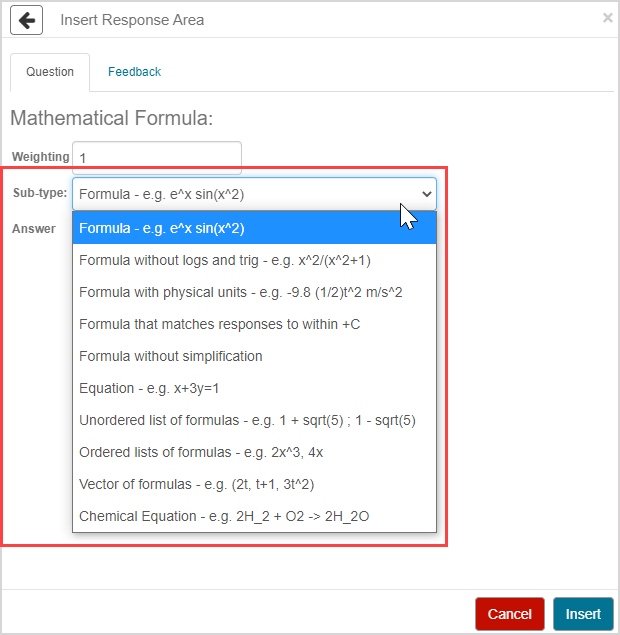# Choose a mathematical formula question sub-type

There are ten sub-types to choose from when authoring a mathematical formula question.Each sub-type has unique syntax requirements of how the Answer field value is defined while authoring.

NOTE: In general, the ten mathematical formula question sub-types accept equivalent responses. However, here are some special notes for equivalent responses and specific sub-types:

• The formula without logs and trig sub-type won't accept logarithmic and trigonometric functions as equivalent responses.
• The formula with physical units sub-type will accept unit equivalents as long as the units are recognized by Möbius.
• The formula that matches responses to within +C sub-type accepts two answers as equivalent if they differ by a constant number.
• The formula without simplification sub-type will only accept unsimplified responses as equivalent responses.
• The equation sub-type will accept any equation that's algebraically equivalent to the correct answer.
• The chemical formula sub-type doesn't accept equivalent responses and requires the student response to be an exact match to the defined correct answer.

TIP: Check out Author a mathematical formula question for full question authoring instructions.

## Formula

The formula sub-type accepts numbers, formulas, and operations, but not equations.

Examples of accepted syntax for defining the Answer field value for this sub-type are:

• (e^x)*sin(x^2)
• 5*x^4

Check out Author a formula sub-type.

## Formula without logs and trig

The formula without logs and trig sub-type accepts numbers and formulas without logarithms or trigonometric functions.

Examples of accepted syntax for defining the Answer field value for this sub-type are:

• 1/sqrt(2)
• (1/3)*3^(1/2)

## Formula with physical units

The formula with physical units sub-type accepts a combination of numeric quantities, algebraic formulas, unit dimensions, and physical units.

Examples of accepted syntax for defining the Answer field value for this sub-type are:

• 6 mg
• 2*t m/s
• sin(x) m/s^2
• (3*t^2+1) km/hr

## Formula that matches responses to within +C

The formula that matches responses to within +C sub-type accepts numbers and formulas as answers within a constant number of the defined correct answer.

Examples of accepted syntax for defining the Answer field value for this sub-type are:

• x*ln(x)
• x*ln(x) +1
• (4/3)*x^3 + (7/5)*x^5 + (11/7)*x^7

## Formula without simplification

The formula without simplification sub-type only accepts the unsimplified form of an expression.

Examples of accepted syntax for defining the Answer field value for this sub-type are:

• 4*x+x
• 3*x^2+9*x+10

## Equation

The equation sub-type accepts responses that use an = symbol.

Examples of accepted syntax for defining the Answer field value for this sub-type are:

• y = 7*x+2
• y = 4*(x+1) +2
• y = 8*(x-2) + 7

Check out Author an equation sub-type.

## Unordered list of formulas

The unordered list of formulas sub-type accepts an unordered list of numbers or formulas separated by semicolons (;).

Examples of accepted syntax for defining the Answer field value for this sub-type are:

• 4; 5*x; 3
• (-5/2)+(1/2)*19^(1/2); (-5/2)-(1/2)*19^(1/2)

## Ordered lists of formulas

The ordered lists of formulas sub-type accepts an ordered list of numbers or formulas separated by commas (,).

Examples of accepted syntax for defining the Answer field value for this sub-type are:

• 2, 4, 8, 16
• sqrt(3)/2, 1/2, sqrt(3)

## Vectors of formulas

The vectors of formulas sub-type is used for questions about Cartesian coordinates or vectors.

Examples of accepted syntax for defining the Answer field value for this sub-type are:

• (2, 5)
• (2, 4*t, 7*t+1)

Check out Author a vector of formulas sub-type.

## Chemical equation

The chemical equation sub-type is used for chemistry questions involving chemical equations.

Examples of accepted syntax for defining the Answer field value for this sub-type are:

• H_2SO_4
• 2H_2+O_2 -> 2H_2O

Check out Author a chemical equation sub-type.